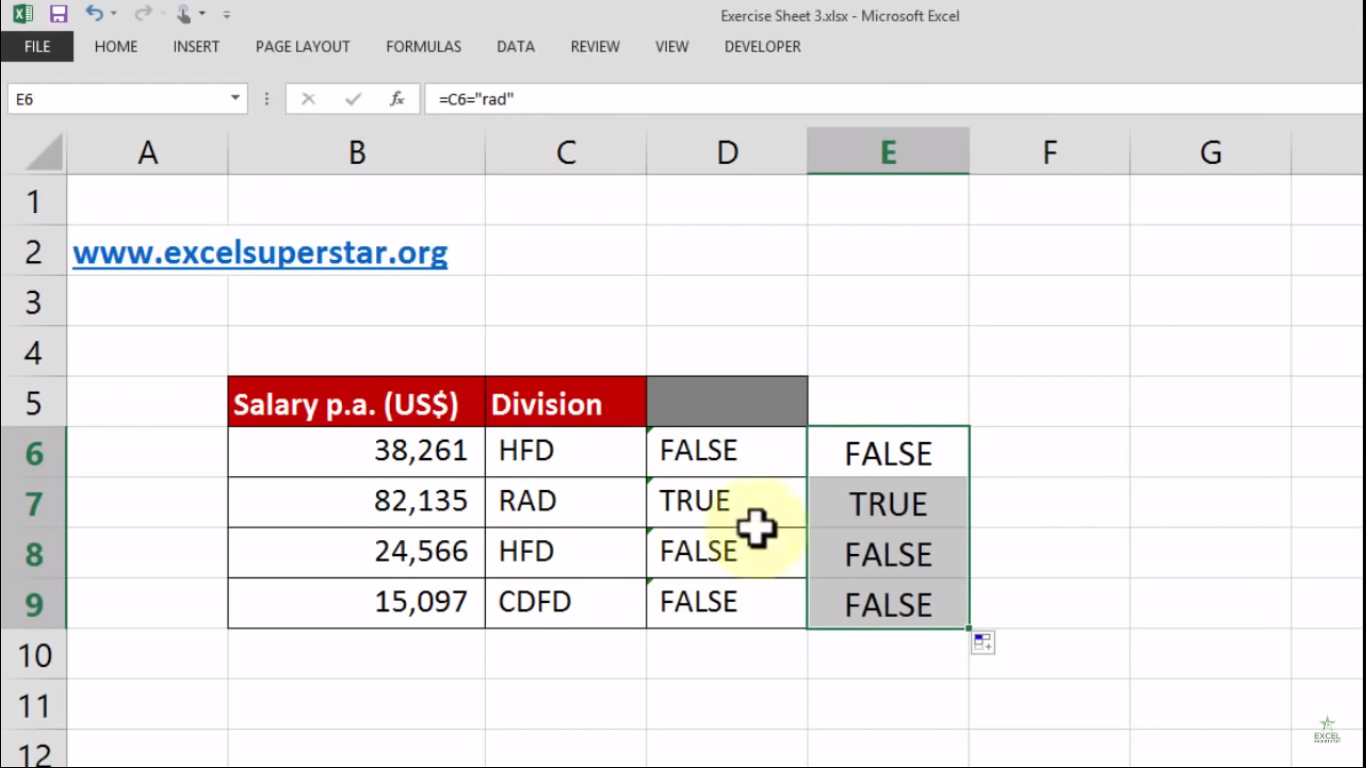# Excel Logical Statements True or FalseIn this tutorial I will be talking about the Foundation of Logical Statements that is true or false in Excel with the help of examples given below:

Question 1: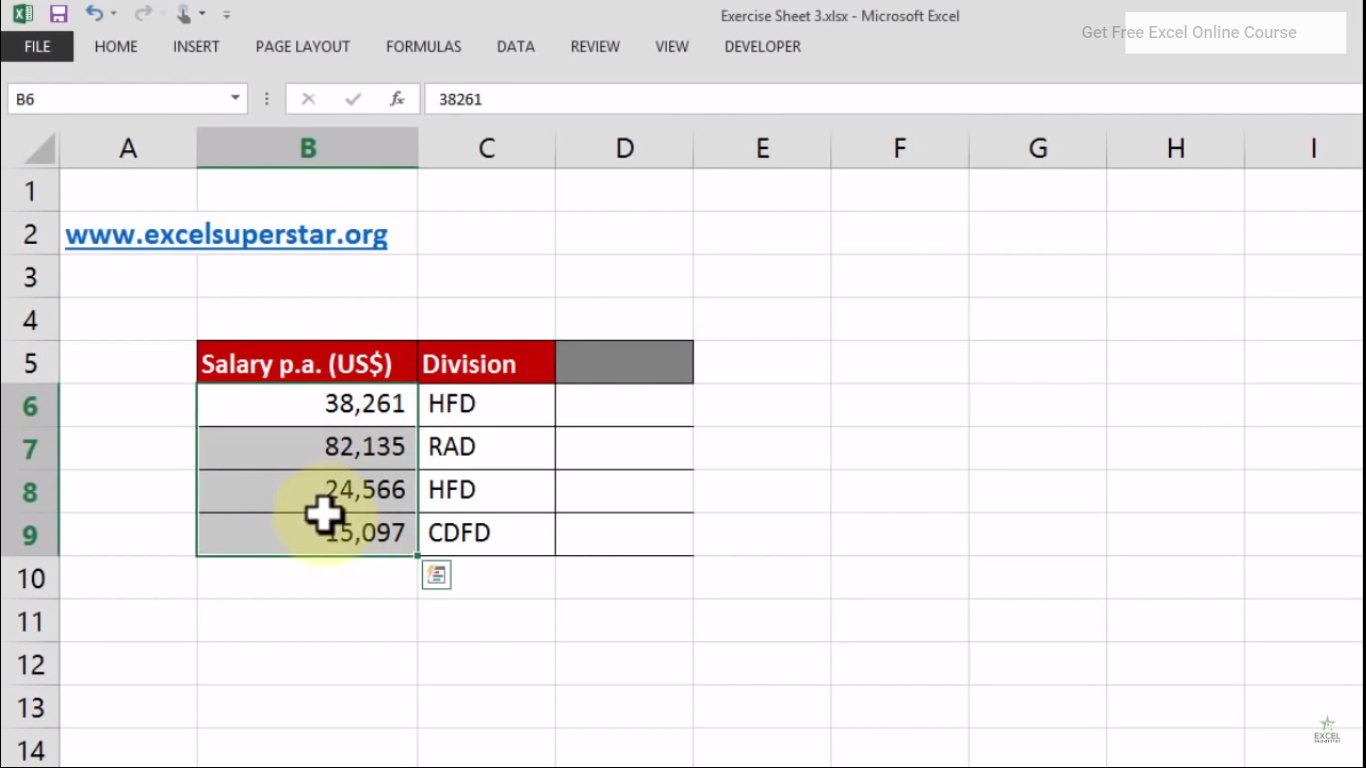In this table, we are having two columns, say salary and division. There are five records mention in the Division column. So now we have to figure out which division is getting a salary higher than \$50,000

So to figure out, we will be applying a Logical statement that is true or false in Excel by following the steps given below:

1. Press = and select the Salary cell =B6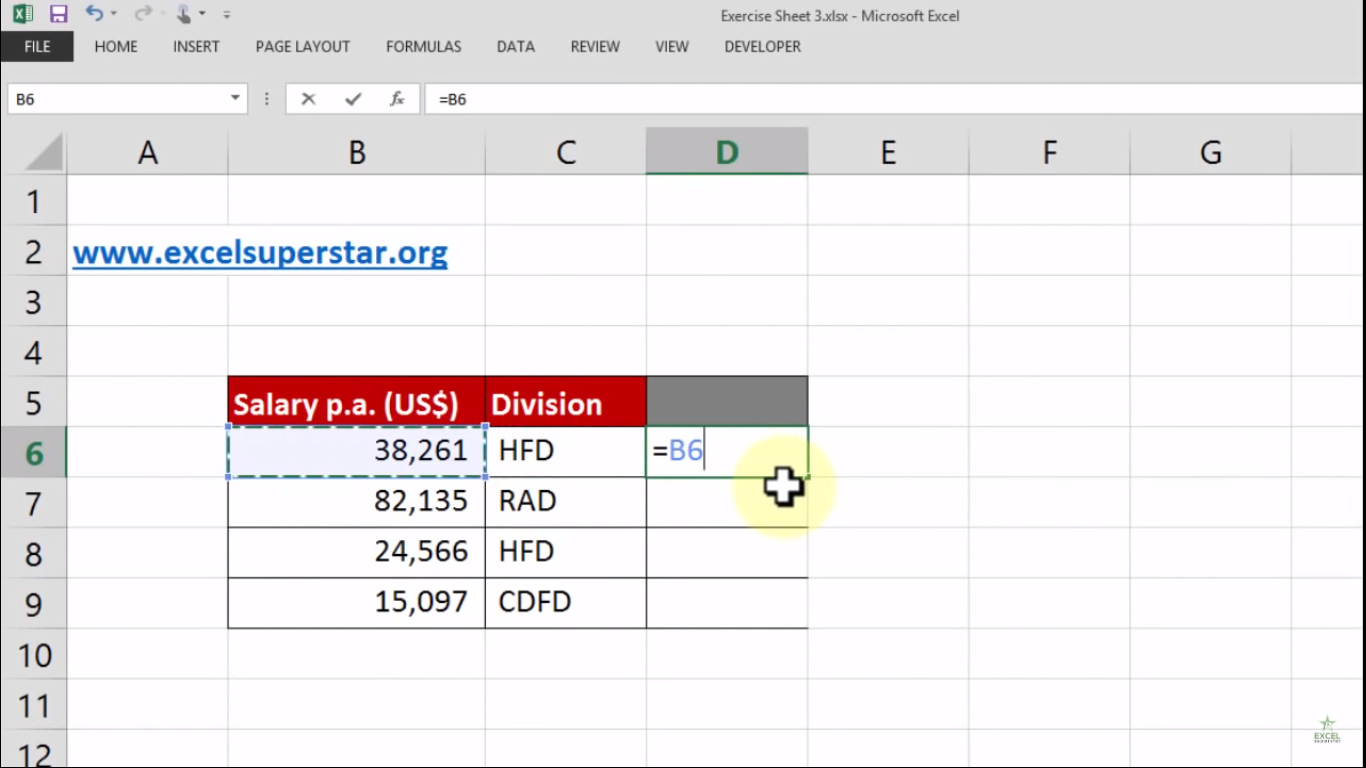2. Write >50000 =B6>50000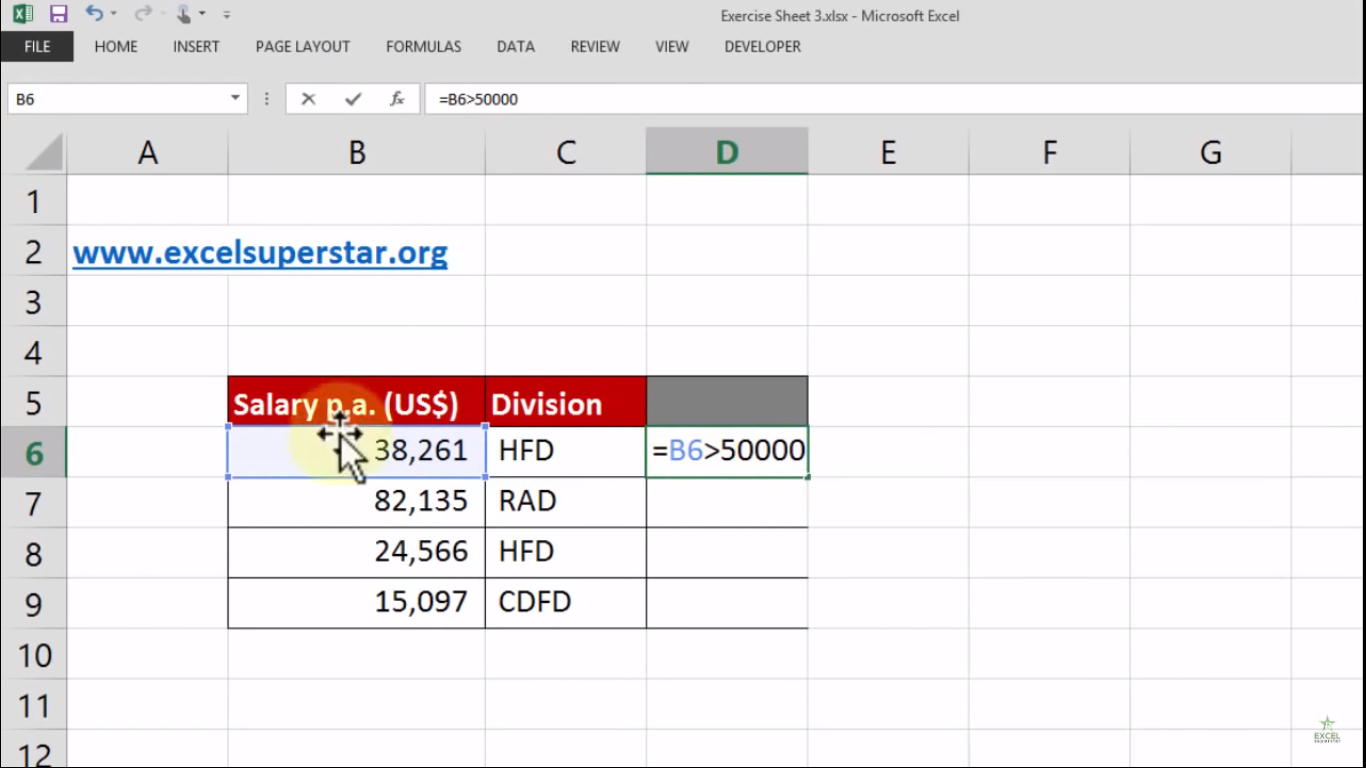3. Press Enter

4. Excel will calculate the answer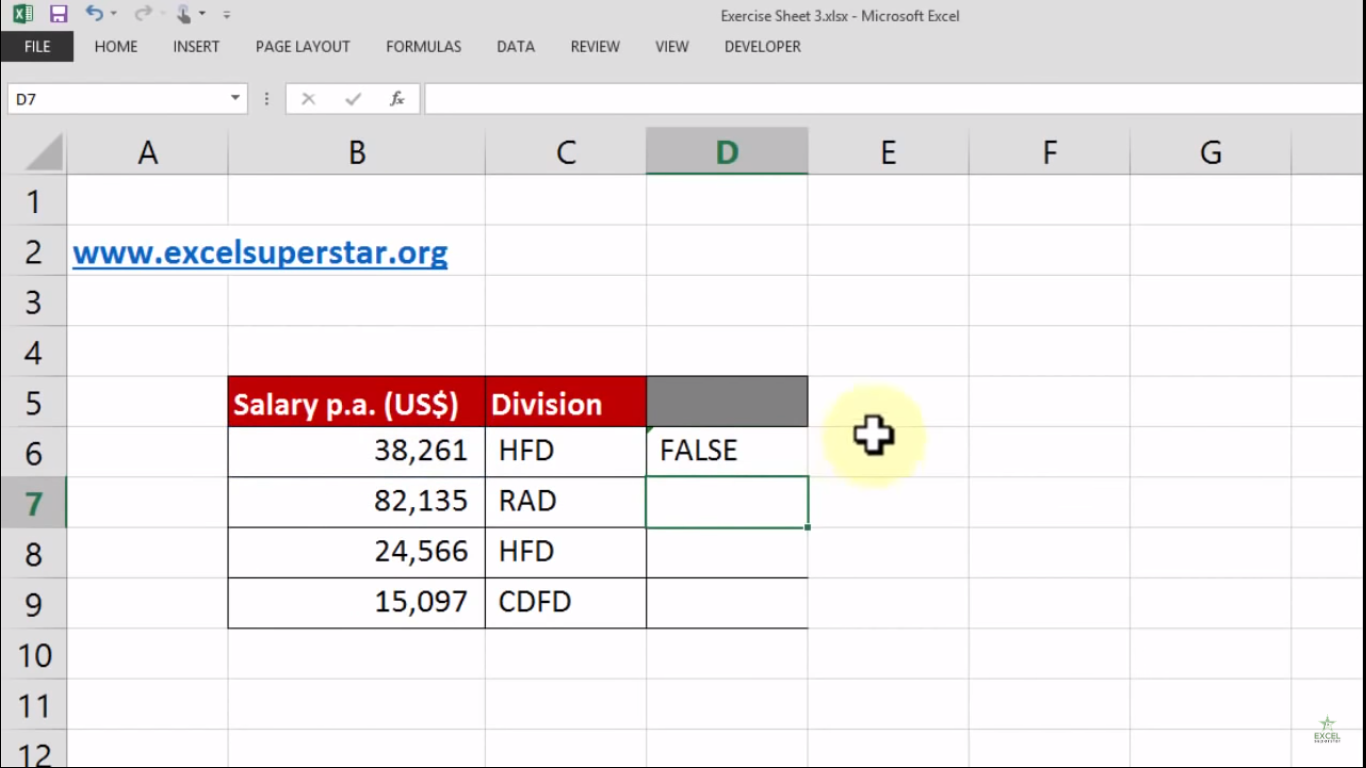5. Lastly, double click on the corner cell to know the other answers in the table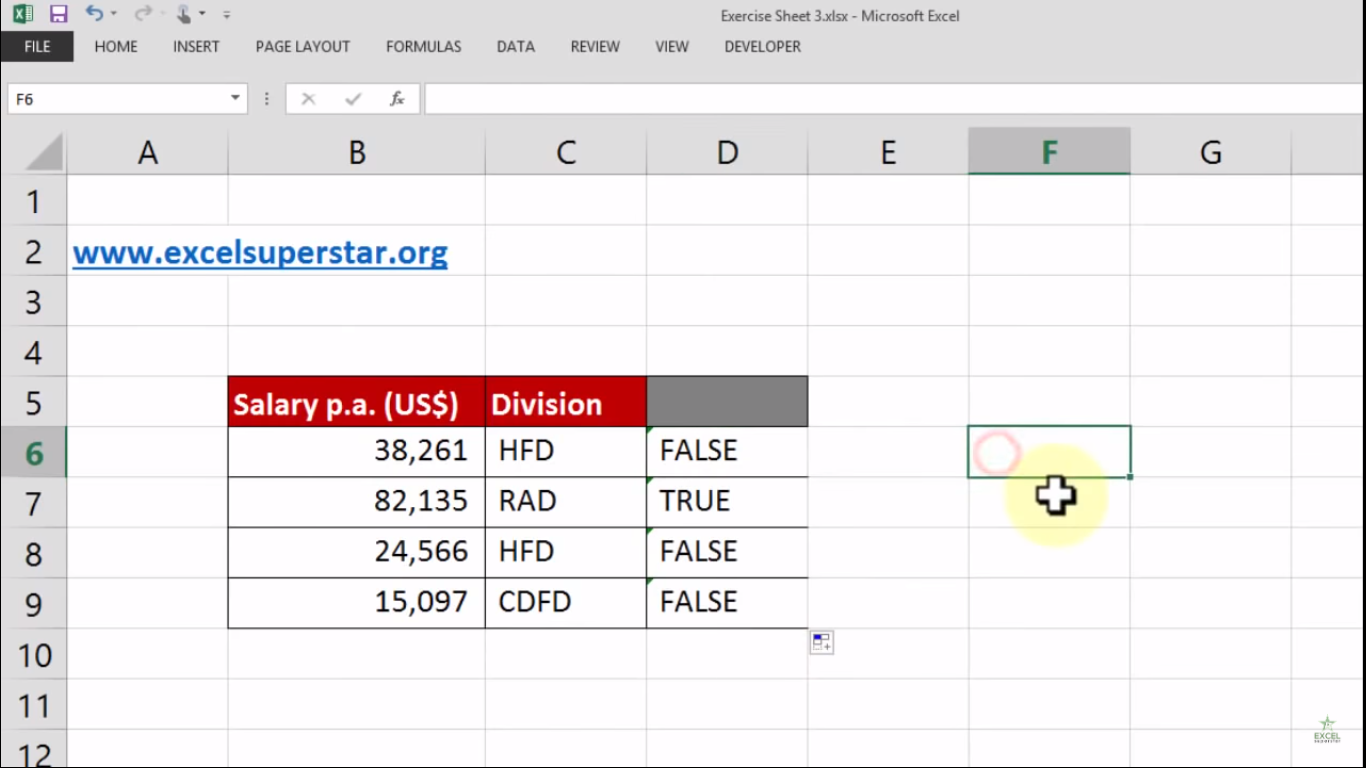Question 2: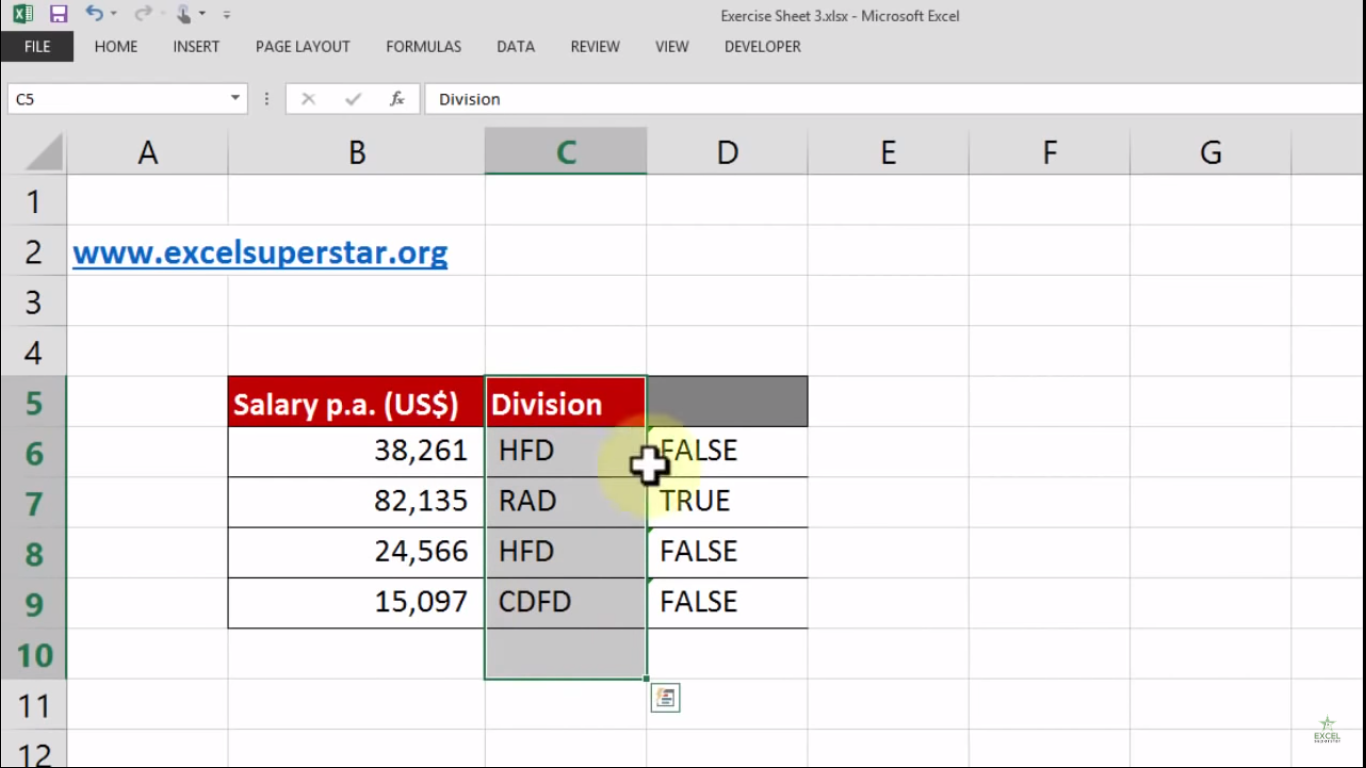In this table, we are having two columns, say salary and division. There are four different Divisions mentioned in the Division column. Now I want to know the total number of persons working in the RAD Division

So in order to find out the number of persons, we will be applying a Logical statement that is true or false in Excel by following the steps given below:

1. Press = and select the Salary cell =C6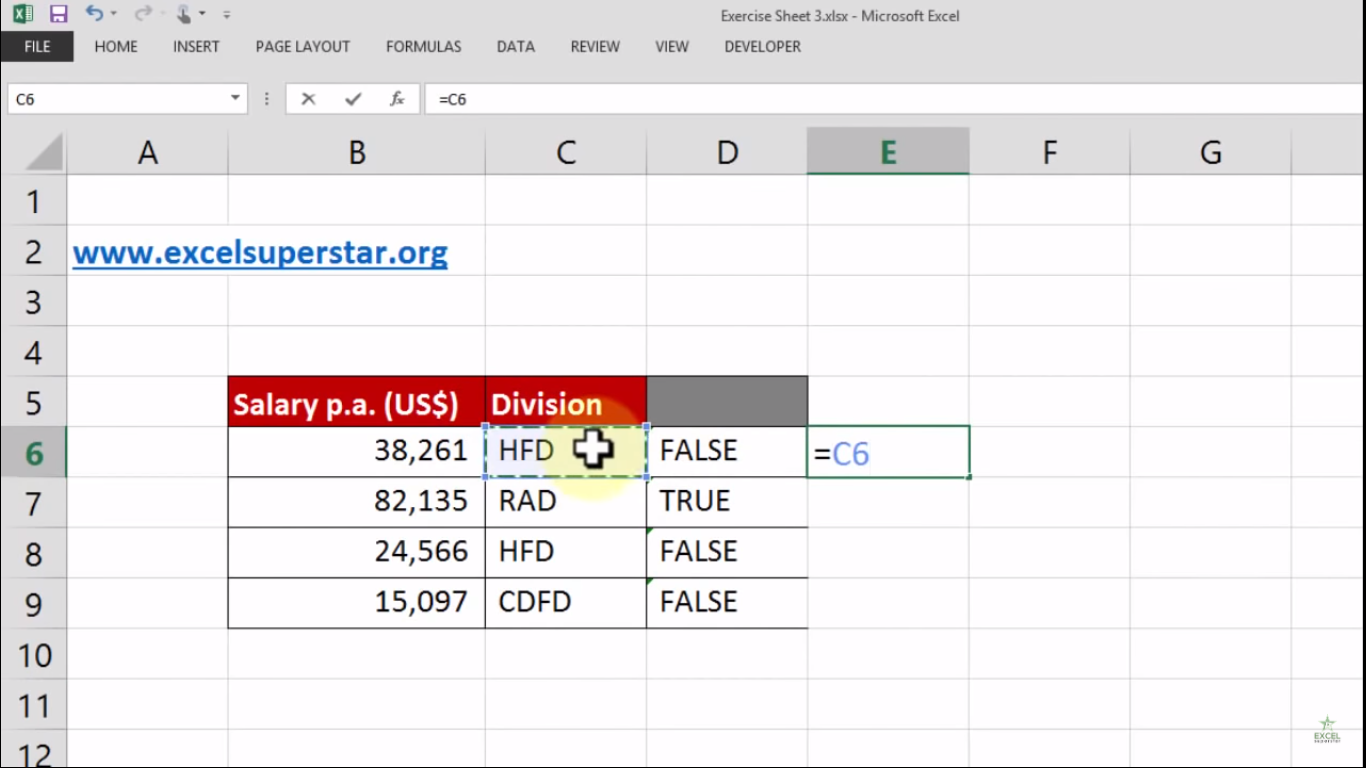2. Again press = and write rad by opening and closing Double Quotation (“) =C6=”rad”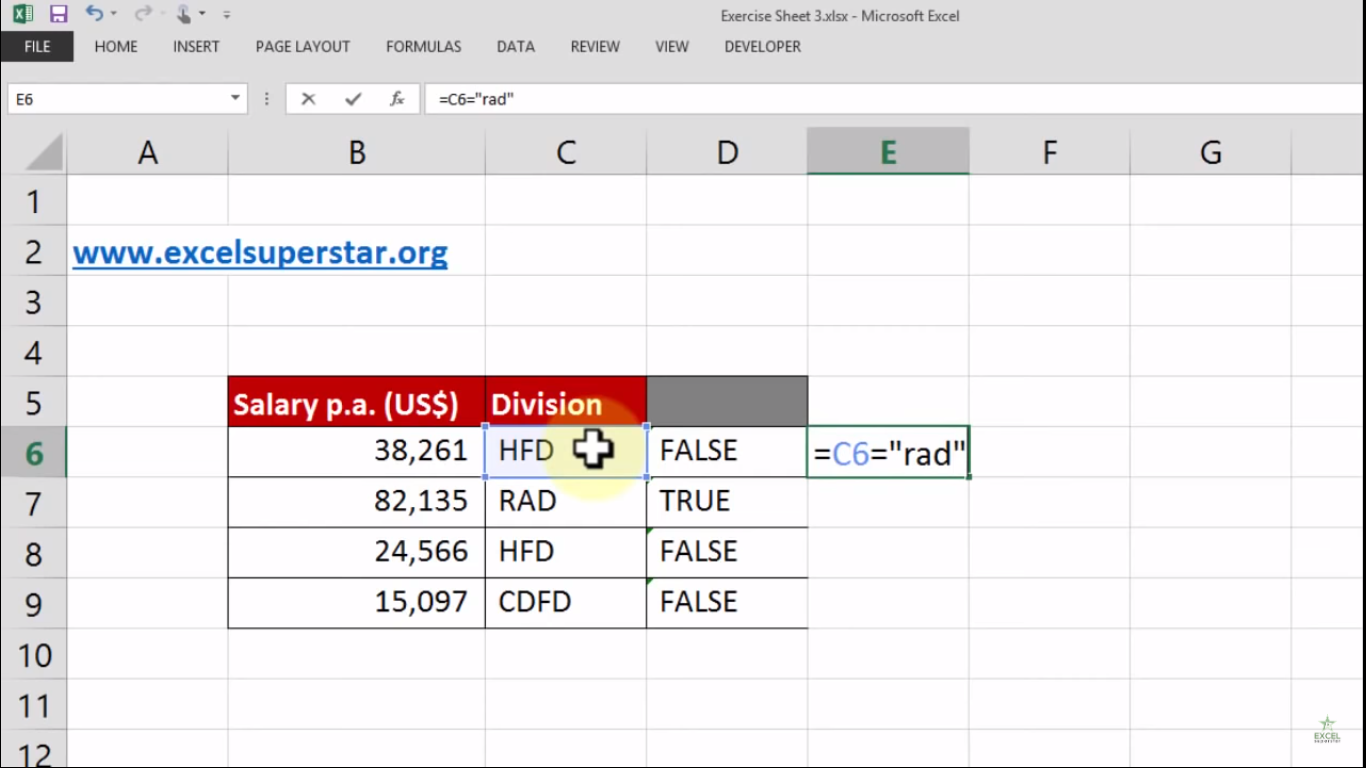Note – This is not case sensitive so you can write in capital or lower letter

3. Press Enter

4. Excel will calculate the answer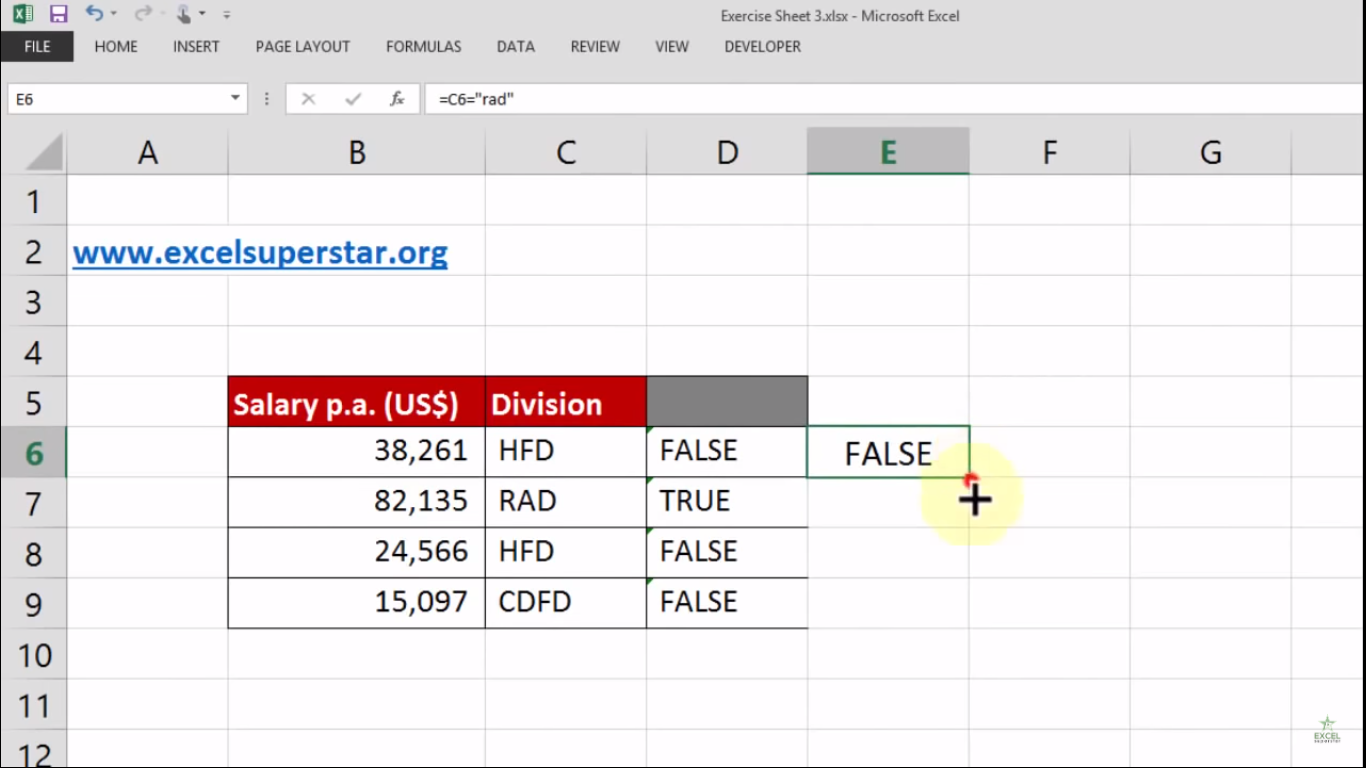5. Lastly, double click on the corner cell to know the other answers in the table